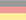# Elementary mathematics

Based on "A Calculus of Number Based on Spatial Forms" by Jeffrey M. James 1993.
```f1(x) = x
f2(x) = -x
f3(x) = ex
f4(x) = ln x
f3(f4(x)) = x   eln x = x
f4(f3(x)) = x   ln ex = x

<>     - Inversion        -0 = 0
() = o - Instanziation    e0 = 1
[] = ∎ - Abstraction    ln 0 = -∞ + [-∞...∞]i

[(a)] = ([a]) = a     Involution                                 Axiom 1     ln ea = eln a = a
(a[bc]) = (a[b])(a[c])  Distribution of a                          Axiom 2     ea + ln (b + c) = ea + ln b + ea + ln c
a<a> =               Inversion of a                             Axiom 3     a - a = 0
<<a>> = a             Inverse Cancellation  (double Inversion)   Theorem 1   -(-a) = a
<a><b> = <ab>          Inverse Collection                         Theorem 2   (-a) + (-b) = -(a + b)

Proof of Inverse Cancellation     for a != ∎
<<a>>
<<a>><a>a   -Inversion of a
a   Inversion of <a>

Proof of Inverse Collection     for a,b != ∎
<a><b>
<a><b>ab<ab>   -Inversion of ab
<ab>   Inversion of a and b

Proof of additive self-inversion of void (ambiguous sign)   -0 = 0
<>
Inversion of void

Cardinality of a (second degree) (Count)
a = ([a]) = ([a][o])             -Involution2, -Involution1
aa = ([a][o])([a][o]) = ([a][oo]) 2x a, -Distribution of [a]
a...a = ([a][o...o])                 induction step to all natural numbers

a * b    =>  ([a][b])
a / b    =>  ([a]<[b]>)     for b !=

Proof of Associativity of multiplication   (a*b)*c = a*(b*c)
([([a]  [b])][c]  )
(  [a]  [b]  [c]  )   Involution1
(  [a][([b]  [c])])   -Involution1

Proof of multiplicative self-inversion of o   1/1 = 1
(<[o]>)
(<   >)   Involution1
(     )   Inversion of void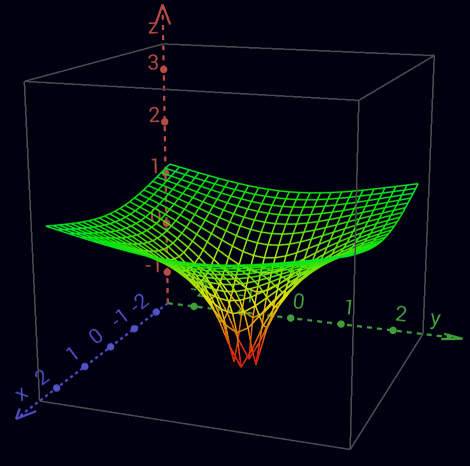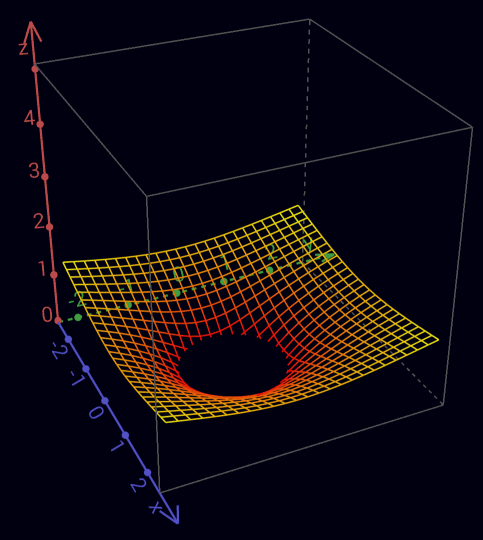Real part of the complex logarithm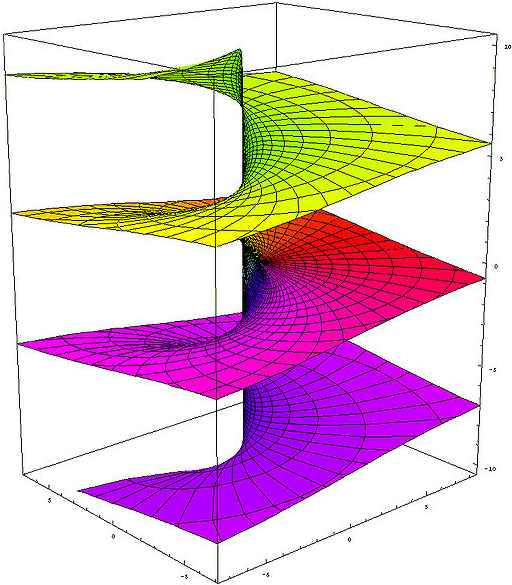Imaginary part of the complex logarithm - Riemann surface

Absorption
([a]∎) =      Zero cardinality of a             Theorem 3   a * 0 = 0
∎ a = ∎    Absorption of a (black hole)      Theorem 4   ∎ + a = ∎

Proof of Zero cardinality
([a]∎)
([a]∎)([a][b])<([a][b])>   -Inversion of ([a][b])
([a][b])<([a][b])>   -Distribution of [a]
Inversion of ([a][b])

Proof of Absorption
∎   a
[(∎ [(a)])]   2x -Involution1
[         ]   Zero cardinality of (a)

Proof of Zero cardinality
([a]∎)
(   ∎)   Absorption of [a]
Involution2

Indefiniteness of cardinality of ∎
∎ = ∎∎ = ∎∎∎ = ∎∎∎∎ = ...    -Absorption of ∎

= <> = [o] = (∎) = [(<>)] = ([<>]) =  <[o]> = <(∎)> 5x Inversion of void, 3x Involution1, 3x Involution2

Indefiniteness of Inversion of ∎
(<∎>) = ([o]<∎>) <= 1/0 is undefined (singularity)

0 * ? = 1   zero don't have multiplicative inverse
0/0 ambiguous
0/a = 0   a/a = 1   a/0 = ∞

inverse Absorption
<∎>  a
<∎><<a>>   -Inverse Cancellation
<∎  <a>>   Inverse Collection
<∎     >   Absorption of <a>

∎<∎>   -Inversion of ∎
=>  Absorption of <∎> or inverse Absorption of ∎
=>  Possibility of arbitrary absorption of everything everywhere
=>  <∎> undefined (Inversion of a black hole is not allowed)
=>  Division by 0 is undefined (singularity)

Cardinality of [a] (third degree)
([a]) = (([[a]])) = (([[a]][o]))                -Involution2, -Involution1
([a][a]) = (([[a]][o])([[a]][o])) = (([[a]][oo]))  2x [a], -Distribution of [[a]]
([a]...[a]) = (([[a]][o...o]))                        induction step to all natural numbers

a     b       Addition                 ooo     ooo       3 + 3             = 6
(   [a]   [b])     Multiplication      (   [ooo]   [ooo])     3 * 3 = 3 + 3 + 3 = 9
((  [[a]]  [b]))    Exponentiation     ((  [[ooo]]  [ooo]))    33    = 3 * 3 * 3 = 27
((( [[[a]]] [b])))   Tetration         ((( [[[ooo]]] [ooo])))   333   = 327       = 7.625.597.484.987
(((([[[[a]]]][b]))))  Hyper-5          (((([[[[ooo]]]][ooo]))))  3...3 7.625.597.484.987 times - our universe is already too small for that
...

ab      =>  (([[a]][b]))
a0 = 1  =>  (([[a]]∎)) = ((∎)) = o                  Absorption of [[a]], Involution2
a1 = a  =>  (([[a]][o])) = (([[a]])) = ([a]) = a    Involution1, 2x Involution2

Inversion
([<a>]b) = <([a]b)>        Inverse Promotion1      Theorem 5   -a * eb = -(a * eb)
(<[<a>]>) = <(<[a]>)>      Inverse Promotion2      Axiom 4     1/-a = -1/a
(<[<a>]>b) = <(<[a]>b)>    Inverse Promotion3      Theorem 6   1/-a * eb = -(1/a * eb)

Proof of Inverse Promotion1     for a != ∎
([<a>]b)
<([a]b)>([a]b)([<a>]b)    -Inversion of ([a]b)
<([a]b)>([a     <a>]b)    -Distribution von b
<([a]b)>([         ]b)    Inversion of a
<([a]b)>([         ] )    Absorption von b
<([a]b)>                  Involution2

Proof of Inverse Promotion3     for a != ∎
(   <[<a>]>   b)
<(<[a]>b)>(  <[a]>  b)(   <[<a>]>   b)    -Inversion of (<[a]>b)
<(<[a]>b)>([(<[a]>)]b)([ (<[<a>]>) ]b)    2x -Involution1
<(<[a]>b)>([(<[a]>)      (<[<a>]>) ]b)    -Distribution von b
<(<[a]>b)>([(<[a]>)     <(<[ a ]>)>]b)    Inverse Promotion2
<(<[a]>b)>([                       ]b)    Inversion of (<[a]>)
<(<[a]>b)>([                       ] )    Absorption von b
<(<[a]>b)>                                Involution2

Proof of irrationality of √2
√2        = a/b
2         = (a/b)2
2 * b2    = (a/b)2 * b2
2 * b * b = a * a

a mod 1 = 0 & b mod 1 = 0 ->
-> (a*a) mod   2 = 0 -> a mod 2 = 0 -> (2*b*b) mod  4 = 0 -> (b*b) mod  2 = 0 -> b mod 2 = 0
-> (a*a) mod   8 = 0 -> a mod 4 = 0 -> (2*b*b) mod 16 = 0 -> (b*b) mod  8 = 0 -> b mod 4 = 0
-> (a*a) mod  32 = 0 -> a mod 8 = 0 -> (2*b*b) mod 64 = 0 -> (b*b) mod 32 = 0 -> b mod 8 = 0
-> (a*a) mod 128 = 0 -> ...
=> √2 is irrational

(([[oo]]<[oo]>)) = ([a]<[b]>)
([[oo]]<[oo]>)  =  [a]<[b]>

b =   o -> a = (([[oo]]<[ oo ]>))        eln 2 /  2
a =   o -> b = (([[oo]]<[<oo>]>))        eln 2 / -2
b =  oo -> a = (([[oo]]<[ oo ]>)[oo])    eln 2 /  2 + ln 2
a =  oo -> b = (([[oo]]<[<oo>]>)[oo])    eln 2 / -2 + ln 2
b = ooo -> a = (([[oo]]<[ oo ]>)[ooo])   eln 2 /  2 + ln 3
a = ooo -> b = (([[oo]]<[<oo>]>)[ooo])   eln 2 / -2 + ln 3
...
=> a and b are not naturally resolvable together
=> (([[oo]]<[oo]>)) is irrational

Operators
-a => <a>                                                                 for a != ∎
a+b => ab
a+b+c => abc
a-b => a<b>                                                                for b != ∎
a*b => ([a][b])
a*b*c => ([a][b][c])
a*-b => ([a][<b>]) = <([a][b])> = ([<a>][b])      +-Inverse Promotion1      for a,b != ∎
a/b => ([a]<[b]>)                                                          for b !=
1/b => ([o]<[b]>) = (<[b]>)                      Involution1               for b !=
a/a => ([a]<[a]>) = o                            Inversion of [a]          for a !=
ab  => (([[a]][b]))
a1  => (([[a]][o])) = (([[a]])) = a              Involution1, 2x Involution2
a-b => (([[a]][<b>]))                                                       for b != ∎
a1/b => (([[a]]<[b]>))    = (([[a]][(<[b]>)]))    -Involution1               for b !=
ac/b => (([[a]]<[b]>[c])) = (([[a]][(<[b]>[c])])) -Involution1               for b !=

abc
(([[a]][(([[b]][c]))]))
(([[a]]  ([[b]][c])  ))   Involution1

((ab)c)1/d = ab*c/d
(([[(([[(([[a]][b]))]][c]))]]<[d]>))
((        [[a]][b]    [c]    <[d]>))   4x Involution1

ab * ac = ab+c
([(([[a]][b]))][(([[a]][c]))])
(  ([[a]][b])    ([[a]][c])  )   2x Involution1
(  ([[a]][b             c])  )   Distribution of [[a]]

ac * bc = (a*b)c
([(([[a]][c]))][(([[b]][c]))])
(  ([[a]][c])    ([[b]][c])  )   2x Involution1
(  ([[a]           [b]][c])  )   Distribution of [c]

1 / e = e-1    =>  ([o]<[(o)]>) = (<[(o)]>) = (<o>)      2x Involution1
1 / e2 = e-2   =>  ([o]<[(oo)]>) = (<[(oo)]>) = (<oo>)   2x Involution1

ln (a*b) = ln a + ln b  =>    [([a][b])]    =   [a][b]     Involution1
ln (a/b) = ln a - ln b  =>    [([a]<[b]>)]  =   [a]<[b]>   Involution1
ln (ab)   = ln a * b     =>  [(([[a]][b]))]  = ([[a]][b])   Involution1

logb a    = ln a/ln b    =>                    ([[a]]<[[b]]>)

1/(1/a) = a
(<[(<[a]>)]>)
(<  <[a]>  >)   Involution1
(    [a]    )   Inverse Cancellation
a         Involution2

(1/a)*(1/b) = 1/(a*b)
([(<  [a]>)][(<[b]  >)])
(  <  [a]>    <[b]  >  )   2x Involution1
(  <  [a]      [b]  >  )   Inverse Collection
(  <[([a]      [b])]>  )   -Involution1

(1/a)b = a-b
( ([[(<[a]>)]][ b ]) )
( ([  <[a]>  ][ b ]) )   Involution1
(<([   [a]   ][ b ])>)   Inverse Promotion1
( ([   [a]   ][<b>]) )   -Inverse Promotion1

a/c + b/d = (a*d + b*c) / c*d
(  [a]          <[c]>)(  [b]          <[d]>)
(  [a][d]  <[d]><[c]>)(  [b][c]  <[c]><[d]>)   -Inversion of [d] and [c]
(  [a][d]  <[d]  [c]>)(  [b][c]  <[c]  [d]>)   2x Inverse Collection
([([a][d])]<[d]  [c]>)([([b][c])]<[c]  [d]>)   2x -Involution1
([([a][d])              ([b][c])]<[c]  [d]>)   -Distribution of <[c][d]>

1/a + 1/b = (a + b) / a*b
(        <[a]>)(        <[b]>)
([b]<[b]><[a]>)([a]<[a]><[b]>)   -Inversion of [b] and [a]
([b]<[b]  [a]>)([a]<[a]  [b]>)   2x Inverse Collection
([ab]<[a][b]>)                   -Distribution of <[a][b]>

(a + b) * (a - b) = a² - b²
([a       b][a                    <b>])
([a       b][a]) ([a           b][<b>])    Distribution of [ab]
([a][a])([b][a]) ([a][<b>])  ([b][<b>])    Distribution of [a] and [<b>]
([a][a])([b][a])<([a][ b ])><([b][ b ])>   2x -Inverse Promotion1
([a][a])                    <([b][ b ])>   Inversion of ([a][b])
(([[a]][oo]))            <(([[b]][oo]))>   Cardinality of [a] and [b]

Numbers
0 =>
1 => o
2 => oo
-1 => <o>
-2 => <oo>
1/2 => (<[oo]>)
2/3 => ([oo]<[ooo]>)
43 => ([b][oooo])ooo             for b = oooooooooo
243 => ([b][([b][oo])oooo])ooo    for b = oooooooooo
1243 => ([b][([b][boo])oooo])ooo   for b = oooooooooo

Arity
{a} = ([oooooooooo][a])   Definition of {}
oooooooooo{a} = {o a}     Carry+            Cardinality of oooooooooo
{a}{b} = {ab}             Collection+
([{a}]b) = {([a]b)}       Promotion+
{} =                      Zero cardinality+

23 * 114 = 2622
([ {oo}ooo ] [ {{o}o}oooo ])
([{oo}][{{o}o}oooo])  ([ooo][{{o}o}oooo])              Distribution of [{{o}o}oooo]
{([ oo ][{{o}o}oooo])} ([ooo][{{o}o}oooo])              Promotion+
{{{o}o}oooo{{o}o}oooo} {{o}o}oooo{{o}o}oooo{{o}o}oooo   2x -Cardinality of {{o}o}oooo
{{{o}o}oooo{{o}o}oooo} {{o}o}{{o}o}{{o}o}oooooooooooo   Collection+ of o
{{{o}o}oooo{{o}o}oooo} {{o}o}{{o}o}{{o}oo}         oo   Carry+
{{{o}o}    {{o}o} {o}{o}{o}  oooooooooooo}         oo   Collection+ of {o}
{{{o}o}    {{o}o} {o}{o}{oo}           oo}         oo   Carry+
{{{o}       {o}      oooooo}           oo}         oo   Collection+ of {{o}}
{{{         oo}      oooooo}           oo}         oo   Collection+ of {{{o}}}

Inverse Arity
/a\ = (<[oooooooooo]>[a])   Definition of /\
/oooooooooo a\ = o/a\       Carry-            Cardinality of oooooooooo
/a\/b\ = /ab\               Collection-
([/a\]b) = /([a]b)\         Promotion-
/\ =                        Zero cardinality-
{/a\} = /{a}\ = a           Arity Cancellation

12,1 * 1,012 = 12,2452
([ {o}oo/o\ ] [ o//o/oo\\\ ])
([{o}][o//o/oo\\\]) ([oo][o//o/oo\\\]) ([/o\][o//o/oo\\\])    2x Distribution of [o//o/oo\\\]
{([ o ][o//o/oo\\\])}([oo][o//o/oo\\\])/([ o ][o//o/oo\\\])\   2x Promotion ±
{       o//o/oo\\\  } o//o/oo\\\o//o/oo\\\    /o//o/oo\\\  \   3x -Cardinality of o//o/oo\\\
{o}{     //o/oo\\\  } o//o/oo\\\o//o/oo\\\    /o//o/oo\\\  \   -Collection+
{o}       /o/oo\\     o//o/oo\\\o//o/oo\\\    /o//o/oo\\\  \   Arity Cancellation1
{o}oo     /o/oo\\      //o/oo\\\ //o/oo\\\    /o//o/oo\\\  \   Collection+ of o
{o}oo     /oo/oo\       /o/oo\\   /o/oo\\       //o/oo\\\  \   Collection- of /o\
{o}oo     /oo/oooo        /oo\      /oo\         /o/oo\\\  \   Collection- of //o\\
{o}oo     /oo/oooo        /ooooo                   /oo\\\  \   Collection- of ///o\\\

100 / 3 = 33,3...
([ {{o}} ]<[ooo]>)
{([  {o}  ]<[ooo]>)}                                                Promotion+
{([oooooooooo]<[ooo]>)}                                             -Carry+
{([ooo]<[ooo]>)([ooo]<[ooo]>)([ooo]<[ooo]>)        ([o]<[ooo]>)}    3x Distribution of <[ooo]>
{(            )(            )(            )        ([o]<[ooo]>)}    3x Inversion of [ooo]
{ooo}                                             {([o]<[ooo]>)}    -Collection+
{ooo}                                            ([{o}]<[ooo]>)     -Promotion+
{ooo}                                     ([oooooooooo]<[ooo]>)     -Carry+
{ooo}([ooo]<[ooo]>)([ooo]<[ooo]>)([ooo]<[ooo]>)    ([o]<[ooo]>)     3x Distribution of <[ooo]>
{ooo}(            )(            )(            )    ([o]<[ooo]>)     3x Inversion of [ooo]
{ooo}ooo                                ([/oooooooooo\]<[ooo]>)     -Carry-
{ooo}ooo                               /([ oooooooooo ]<[ooo]>) \   Promotion-
{ooo}ooo/([ooo]<[ooo]>)([ooo]<[ooo]>)([ooo]<[ooo]>)([o]<[ooo]>) \   3x Distribution of <[ooo]>
{ooo}ooo/(            )(            )(            )([o]<[ooo]>) \   3x Inversion of [ooo]
{ooo}ooo/ooo                            ([/oooooooooo\]<[ooo]>) \   -Carry-
{ooo}ooo/ooo                           /([ oooooooooo ]<[ooo]>)\\   Promotion-
...

100 / 3,3 = 30,3030...
([ {{o}} ]<[ooo/ooo\]>)
{([  {o}  ]<[ooo/ooo\]>)}                                                 Promotion+
{([oooooooooo]<[ooo/ooo\]>)}                                              -Carry+
{([ooooooooo/ooo\/ooo\/ooo\/o\]<[ooo/ooo\]>)}                             -Carry- and 3x -Collection-
{([ooo/ooo\]<[ooo/ooo\]>)(dito)(dito)        ([ /o\ ]<[ooo/ooo\]>)}       3x Distribution of <[ooo/ooo\]>
{(                      )(    )(    )        ([ /o\ ]<[ooo/ooo\]>)}       3x Inversion of [ooo/ooo\]
{ooo}                                       {([ /o\ ]<[ooo/ooo\]>)}       -Collection+
{ooo}                                        ([{/o\}]<[ooo/ooo\]>)        -Promotion+
{ooo}                                        ([  o  ]<[ooo/ooo\]>)        Arity Cancellation1
{ooo}                                 ([/oooooooooo\]<[ooo/ooo\]>)        -Carry-
{ooo}                                /([ oooooooooo ]<[ooo/ooo\]>)    \   Promotion-
{ooo}                 /([ooooooooo/ooo\/ooo\/ooo\/o\]<[ooo/ooo\]>)    \   -Carry- and 3x -Collection-
{ooo}/([ooo/ooo\]<[ooo/ooo\]>)(dito)(dito)     ([/o\]<[ooo/ooo\]>)    \   3x Distribution of <[ooo/ooo\]>
{ooo}/(                      )(    )(    )     ([/o\]<[ooo/ooo\]>)    \   3x Inversion of [ooo/ooo\]
{ooo}/ooo                           ([//oooooooooo\\]<[ooo/ooo\]>)    \   -Carry-
{ooo}/ooo                         //([  oooooooooo  ]<[ooo/ooo\]>)  \\\   2x Promotion-
{ooo}/ooo            //([ooooooooo/ooo\/ooo\/ooo\/o\]<[ooo/ooo\]>)  \\\   -Carry- and 3x -Collection-
{ooo}/ooo//([ooo/ooo\]<[ooo/ooo\]>)(dito)(dito)([/o\]<[ooo/ooo\]>)  \\\   3x Distribution of <[ooo/ooo\]>
{ooo}/ooo//(                      )(    )(    )([/o\]<[ooo/ooo\]>)  \\\   3x Inversion of [ooo/ooo\]
{ooo}/ooo//ooo                      ([//oooooooooo\\]<[ooo/ooo\]>)  \\\   -Carry-
{ooo}/ooo//ooo                    //([  oooooooooo  ]<[ooo/ooo\]>)\\\\\   2x Promotion-
...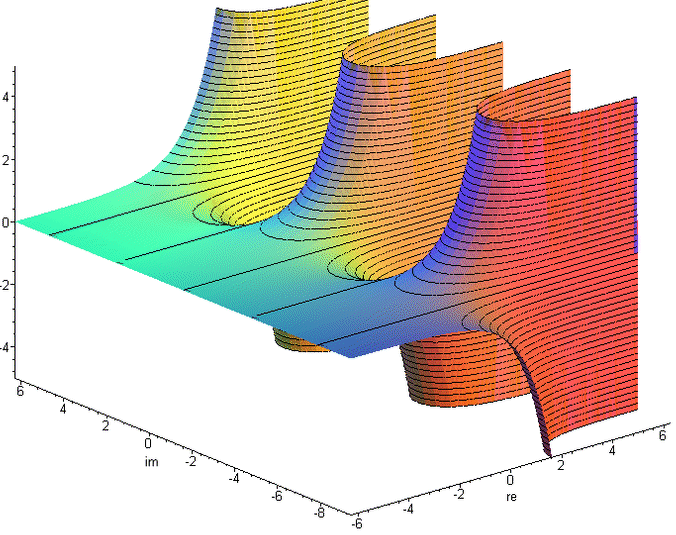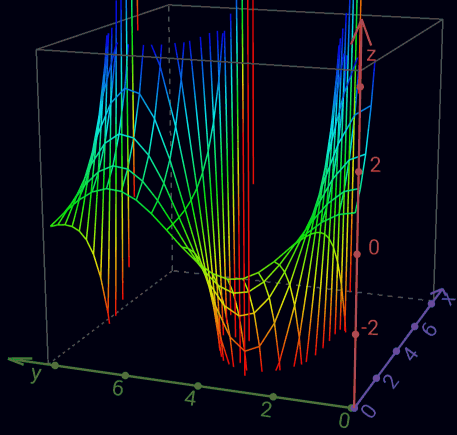Real part of the complex exponential function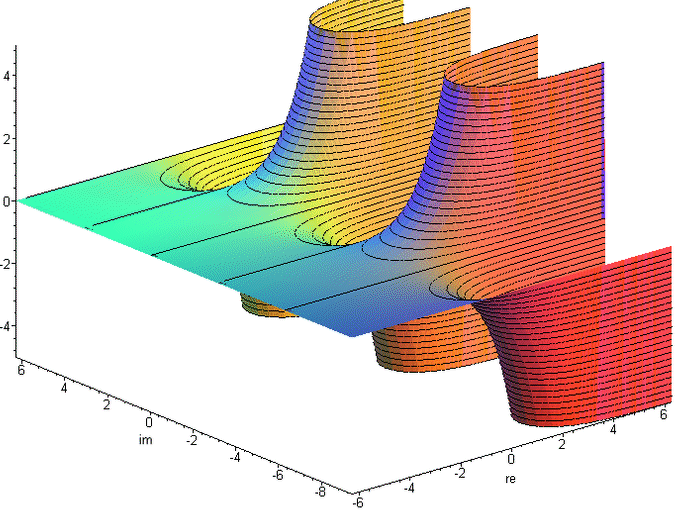Imaginary part of the complex exponential function

Transcendental
J  =  [<o>]                Phase Element            Definition   ln -1 = πi              (~3.1415926535i)
(J) = ([<o>]) = <o>         Involution2                           eJ = eπi = -1
(o)                         Euler number                          e1 = e                  (~2.71828182845)
((o))                                                             ee                      (~15.1542622414)
[<(a)>] = a[<o>]            Phase Independence of a  Theorem 7    ln -ea = a + ln -1
(a  JJ ) = (a)              J-cancellation1          Theorem 8    ea + 2*ln -1 = ea + ln (-1)² = ea + ln 1 = ea = ea + 2πi
(a<bJJ>) = (a<b>)           J-cancellation2          Theorem 9    ea - b - 2*ln -1 = ea - b - ln (-1)² = ea - b - ln 1 = ea - b = ea - b - 2πi
(J) = (<J>)                 Self-Inversion of J      Theorem 10   eJ = e-J
(([J]<[oo]>))               i and <i>                             eJ/2 =  √-1 = ±i
i = (<[<i>]>) = <(<[i]>)>   imaginary unit           Theorem 11   i = 1/-i = -e-ln i
([<J>][i]) = ([J][<i>])     Circle number Pi                      -J*i = J/i = J*-i = π   (~3.1415926535)

Like ln in the imaginary, by an arbitrary multiple of 2π is ambiguous, so at e all values are repeated every 2πi.
To represent this, the J-cancellation and self-inversion of J is permissible only within an instantiation,
or they must be contained in at least one more instantiation than in abstractions.

Proof of Phase Independence of a     for a != [∎]
[<(a)>              ]
[<(a)>( [         ])]  -Involution2
[<(a)>(a[         ])]  -Absorption of a
[<(a)>(a[o     <o>])]  -Inversion of o
[<(a)>(a[o])(a[<o>])]  Distribution of a
[<(a)>(a   )(a[<o>])]  Involution1
[           (a[<o>])]  Inversion of (a)
a[<o>]    Involution1

Proof of J-cancellation     within ()
[<o>][<o>]
[<([<o>])>]   -Phase Independence von [<o>]
[<  <o>  >]   Involution2
[    o    ]   Inverse Cancellation
Involution1

Proof of J-cancellation1     within ()
(a[<o>][<o>])      -Involution1
<<(a[ o ][ o ])>>    2x Inverse Promotion1
(a[ o ][ o ])      Inverse Cancellation
(a          )      2x Involution1

Proof of Self-Inversion of J     within ()
(J      )
(J<    >)   -Inversion of void
(J<J  J>)   -J-cancellation2
(J<J><J>)   -Inverse Collection
(    <J>)   Inversion of J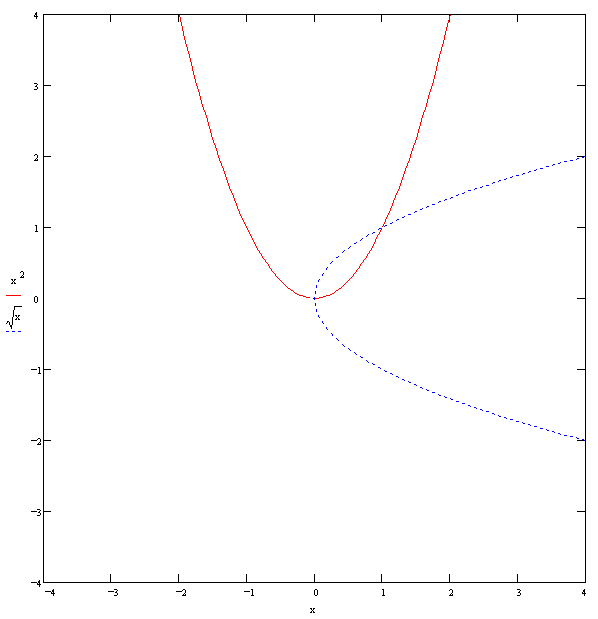f1(x) = x2
f2(x) = √x
f1(f2(x)) = x   (√x)2 = x
f2(f1(x)) = ±x   √(x2) = ±xReal part and imaginary part of the complex square root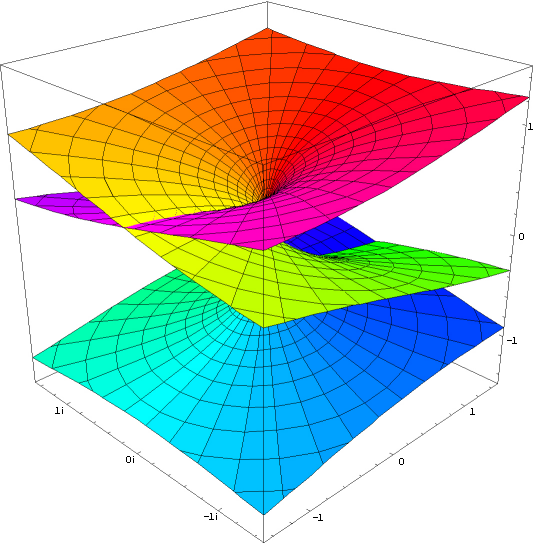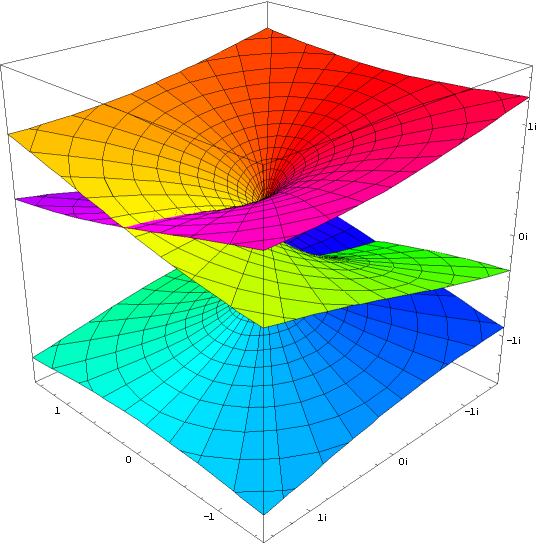Real part and imaginary part of the complex cube root

Proof of ambiguity of (([J]<[oo]>)) or √-1
Whenever the exponent is a non-integer rational number, there is ambiguity.
And if the exponent is less than or equal to 0, the base equals 0 gives a degenerate point.

i * i = -i * -i = (±i)2 = -1
([(([J]<[oo]>))][(([J]<[oo]>))])
(([[(([J]<[oo]>))]][oo]))       Cardinality of [(([J]<[oo]>))]
((    [J]<[oo]>    [oo]))       2x Involution1
((    [J]              ))       Inversion of [oo]
<o>                       2x Involution2
and
([<(([J]<[oo]>))>][<(([J]<[oo]>))>])
<<([ (([J]<[oo]>)) ][ (([J]<[oo]>)) ])>>   2x Inverse Promotion1
([ (([J]<[oo]>)) ][ (([J]<[oo]>)) ])     Inverse Cancellation
(([[(([J]<[oo]>))]][oo])           )     Cardinality of [(([J]<[oo]>))]
((    [J]<[oo]>    [oo])           )     2x Involution1
((    [J]              )           )     Inversion of [oo]
<o>                                2x Involution2

Proof of imaginary unit1
(<[<<(([ J ]<[oo]>))>>]>)
(<    ([ J ]<[oo]>)    >)   Inverse Cancellation, Involution1
(     ([<J>]<[oo]>)     )   -Inverse Promotion1
(     ([ J ]<[oo]>)     )   -Self-Inversion of J

Proof of imaginary unit2
<(<[(([ J ]<[oo]>))]>)>
<(<  ([ J ]<[oo]>)  >)>   Involution1
<(   ([<J>]<[oo]>)   )>   -Inverse Promotion1
<(   ([ J ]<[oo]>)   )>   -Self-Inversion of J

-1 * -1 = 1
([<o>][<o>])
(          )   J-cancellation1

ee = ee
(([[(o)]][(o)]))
((         o  ))   3x Involution1

<  a  >  = ([<([a])>]) = ([a][<o>]) = ([a]J)   2x-Involution2, Phase Independence of [a]
[<  a  >] =  [<([a])>]  =  [a][<o>]  =  [a]J    -Involution2, Phase Independence of [a]
<( a )>  = ([<( a )>]) =   (a[<o>]) =   (aJ)   -Involution2, Phase Independence von  a

Indefiniteness of Cardinality of J     within ()
... = (<JJJJ>) = (<JJ>) =  o  = (JJ) = (JJJJ) = ...     J-cancellation1, J-cancellation2, Inversion of void
... = (<JJJ>) = (<J>) = (J) = (JJJ) = (JJJJJ) = ...   J-cancellation1, J-cancellation2, Self-Inversion of J

Indefiniteness of [∎]     within ()
(   [∎]    )
(   [∎]  JJ)   -J-cancellation1
([<([∎])>]J)   -Phase Independence of [∎]
([<  ∎  >]J)   Involution2 - Indefiniteness der Inversion of ∎

Indefiniteness of 00
(([∎]∎))
((   ∎))   Absorption of [∎]
(      )   Involution2
=> 00 = 1 but from the Indefiniteness of [∎] follows in principle the Indefiniteness of 00

a0 = 1
(([[a]]∎))
((   ∎))   Absorption of [[a]]
(      )   Involution2

0n = 0   n ∈ ℕ
(([∎][o...o]))
(∎...∎)   Cardinality of ∎
(∎    )   Absorption of ∎
Involution2

ln 0 + ln -1 = ln 0
∎J
[<(∎)>]   -Phase Independence of ∎
[<   >]   Involution2
[     ]   Inversion of void

Proof of multiplicative Self-Inversion of (([J]<[oo]>)) (like o)
1/±i = ∓i
(<[(([ J ]<[oo]>))]>)
(<  ([ J ]<[oo]>)  >)   Involution1
(   ([<J>]<[oo]>)   )   -Inverse Promotion1
(   ([ J ]<[oo]>)   )   -Self-Inversion of J
and
(<[<(([ J ]<[oo]>))>]>)
<(<[ (([ J ]<[oo]>)) ]>)>   -Inverse Promotion2
<(<   ([ J ]<[oo]>)   >)>   Involution1
<(    ([<J>]<[oo]>)    )>   -Inverse Promotion1
<(    ([ J ]<[oo]>)    )>   -Self-Inversion of J

∓i = ±i
<(([ J ]<[oo]>))>
(<[<<(([ J ]<[oo]>))>>]>)   imaginary unit11
(<    ([ J ]<[oo]>)    >)   Inverse Cancellation, Involution1
(     ([<J>]<[oo]>)     )   -Inverse Promotion1
(     ([ J ]<[oo]>)     )   -Self-Inversion of J

±i = ∓i
(([ J ]<[oo]>))
<(<[(([ J ]<[oo]>))]>)>   imaginary unit12
<(<  ([ J ]<[oo]>)  >)>   Involution1
<(   ([<J>]<[oo]>)   )>   -Inverse Promotion1
<(   ([ J ]<[oo]>)   )>   -Self-Inversion of J

J/i = i*π / i = π
([J]<[(([ J ]<[oo]>))]>)
([J]<  ([ J ]<[oo]>)  >)   Involution1
([J]   ([<J>]<[oo]>)   )   -Inverse Promotion1
([J]   ([ J ]<[oo]>)   )   -Self-Inversion of J
and
([ J ]<[<(([ J ]<[oo]>))>]>)
<([ J ]<[ (([ J ]<[oo]>)) ]>)>   Inverse Promotion3
([<J>]<[ (([ J ]<[oo]>)) ]>)    -Inverse Promotion1
([<J>]<   ([ J ]<[oo]>)   >)    Involution1
([<J>]    ([<J>]<[oo]>)    )    -Inverse Promotion1
([<J>]    ([ J ]<[oo]>)    )    -Self-Inversion of J

J * -i = π
([ J ][<(([ J ]<[oo]>))>])
<([ J ][ (([ J ]<[oo]>)) ])>   Inverse Promotion1
([<J>][ (([ J ]<[oo]>)) ])    -Inverse Promotion1
([<J>]   ([ J ]<[oo]>)   )    Involution1
and
([J][<<(([ J ]<[oo]>))>>])
([J][  (([ J ]<[oo]>))  ])    Inverse Cancellation
([J]    ([ J ]<[oo]>)    )    Involution1

π * i = J
([([<J>][i])][i])
(  [<J>][i]  [i])     Involution1
(  [<J>][<o>   ])     i * i = -1
<<(  [ J ][ o    ])>>   2x Inverse Promotion1
J                Inverse Cancellation, Involution1, 2x Involution2

ii = eJ*i/2 = e-π/2 = e-π/2-2πk  k ∈ ℤ
(([[(([J]<[oo]>))]][(([J]<[oo]>))]))
((    [J]<[oo]>      ([J]<[oo]>)  ))   3x Involution1
and
( ([[(([ J ]<[oo]>))]][<(([J]<[oo]>))>]) )
(<(    [ J ]<[oo]>    [ (([J]<[oo]>)) ])>)   2x Involution1, Inverse Promotion1
( (    [<J>]<[oo]>    [ (([J]<[oo]>)) ]) )   -Inverse Promotion1
( (    [ J ]<[oo]>       ([J]<[oo]>)   ) )   Involution1, -Self-Inversion of J

i-2i = eπ
(([[(([J]<[oo]>))]][(([J]<[oo]>))][oo]))
((    [J]<[oo]>      ([J]<[oo]>)  [oo]))   3x Involution1
((    [J]            ([J]<[oo]>)      ))   Inversion of [oo]
and
( ([[(([ J ]<[oo]>))]][<(([J]<[oo]>))>][oo]) )
(<(    [ J ]<[oo]>    [ (([J]<[oo]>)) ][oo])>)   2x Involution1, Inverse Promotion1
( (    [<J>]<[oo]>    [ (([J]<[oo]>)) ][oo]) )   -Inverse Promotion1
( (    [ J ]             ([J]<[oo]>)       ) )   Involution1, -Self-Inversion of J

1/√a = √(1/a)
(<[(([ [a] ]<[oo]>))]>)
(<  ([ [a] ]<[oo]>)  >)   Involution1
(   ([<[a]>]<[oo]>)   )   -Inverse Promotion1

√9 = √±32 = ±3
(([[   ooooooooo   ]]<[oo]>))
(([[(  [ooo][ooo] )]]<[oo]>))   Cardinality of ooo
(([[(([[ooo]][oo]))]]<[oo]>))   Cardinality of [ooo]
((    [[ooo]][oo]    <[oo]>))   2x Involution1
((    [[ooo]]              ))   Inversion of [oo]
ooo                     2x Involution2
and
(([[       ooooooooo      ]]<[oo]>))
(([[  (  [ ooo ][ ooo ])  ]]<[oo]>))   Cardinality of ooo
(([[<<(  [ ooo ][ ooo ])>>]]<[oo]>))   -Inverse Cancellation
(([[  (  [<ooo>][<ooo>])  ]]<[oo]>))   2x -Inverse Promotion1
(([[  (([[<ooo>]][oo]) )  ]]<[oo]>))   Cardinality of [<ooo>]
((      [[<ooo>]][oo]       <[oo]>))   2x Involution1
((      [[<ooo>]]                 ))   Inversion of [oo]
<ooo>                        2x Involution2

-22 = 22
(([[<oo>]][oo]))
([<oo>][<oo>])     -Cardinality of [<oo>]
<<([ oo ][ oo ])>>   2x Inverse Promotion1
([ oo ][ oo ])     Inverse Cancellation
(([[oo]][oo]))       Cardinality of [oo]

ab = -ab
((      [[ a ]]                 [b]))
((      [[ a ]][oo]       <[oo]>[b]))   -Inversion of [oo]
(([[  (([[ a ]][oo]))  ] ]<[oo]>[b]))   2x -Involution1
(([[  (  [ a ][ a ] )  ] ]<[oo]>[b]))   -Cardinality of [a]
(([[<<(  [ a ][ a ] )>>] ]<[oo]>[b]))   -Inverse Cancellation
(([[  (  [<a>][<a>] )  ] ]<[oo]>[b]))   2x -Inverse Promotion
(([[  (([[<a>]][oo]))  ] ]<[oo]>[b]))   Cardinality of [<a>]
((      [[<a>]][oo]       <[oo]>[b]))   2x Involution1                      !!! ambiguity
((      [[<a>]]                 [b]))   Inversion of [oo]

Self-Inversion   problem
-0 = 0
1/1 = 1
<∎>
∎ = ∎∎ = ∎∎∎ = ∎∎∎∎ = ...
J = <J>            ... = <JJJJ> = <JJ> =   = JJ = JJJJ = ... , ambiguity of (([J]<[oo]>))
1/±i = ∓i
ab = -abThree-dimensional representation of the Eulerian formula ez*i = cos z + i sin z   =>  (([z]([J]<[oo]>)))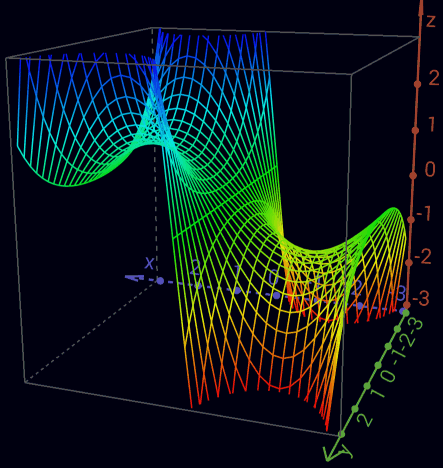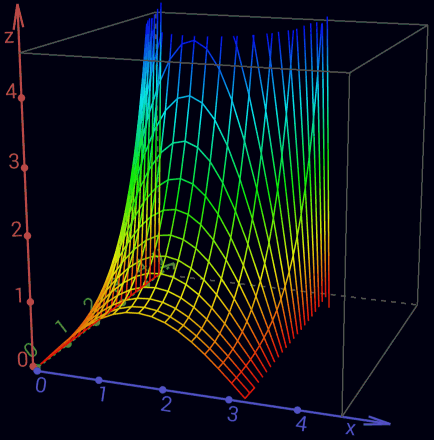Real part of the complex sine function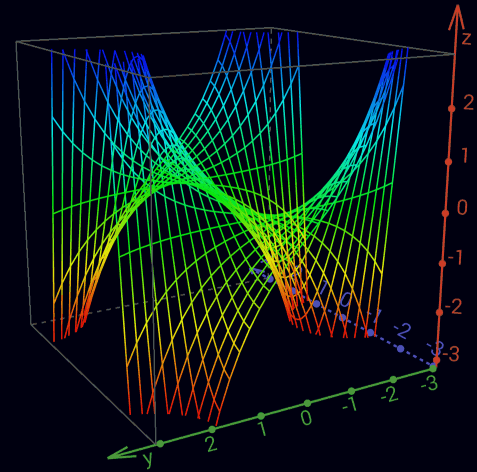Imaginary part of the complex sine function

an+1 =  ([an]J)      o -> (J) -> o -> (J) -> o -> ...  <=  (1 -> -1 ->  1 -> -1 -> 1 -> ...)
an+1 =  ([an][i])    o -> i -> (J) -> <i> -> o -> ...  <=  (1 ->  i -> -1 -> -i -> 1 -> ...)
an+1 = <([an][i])>   o -> <i> -> (J) -> i -> o -> ...  <=  (1 -> -i -> -1 ->  i -> 1 -> ...)

ea*i = cos a + i sin a    =>     (([a][i]))
e-a*i = cos a - i sin a   =>                 (([<a>][i]))
sin a = (ea*i - e-a*i)/2i  =>  ([ (([a][i]))<(([<a>][i]))>]<[oo][i]>)
cos a = (ea*i + e-a*i)/2   =>  ([ (([a][i])) (([<a>][i])) ]<[oo]   >)

ea  = cosh a + sinh a   =>     (a)
e-a = cosh a - sinh a   =>         (<a>)
sinh a = (ea - e-a)/2   =>   ([(a)<(<a>)>]<[oo]>)
cosh a = (ea + e-a)/2   =>   ([(a) (<a>) ]<[oo]>)

sin(x + i y) = sin(x) cosh(y) + i cos(x) sinh(y)
cos(x + i y) = cos(x) cosh(y) - i sin(x) sinh(y)
sin(z) = -i sinh(i z)
sinh(z) =  -i sin(i z)
cos(z) =    cosh(i z)
cosh(z) =     cos(i z)
sin'(z) =  cos(z)
sinh'(z) = cosh(z)
cos'(z) = -sin(z)
cosh'(z) = sinh(z)

unit circle    x2 + y2 = 1   (([[x]][oo])) (([[y]][oo]))  = o
unit hyperbole x2 - y2 = 1   (([[x]][oo]))<(([[y]][oo]))> = o

```